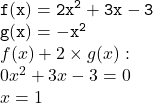## URGENT HELP Find the points of intersection of the graphs involving the following pair of functions. f(x)=2x^2 + 3x

Question

URGENT HELP

Find the points of intersection of the graphs involving the following pair of functions.

f(x)=2x^2 + 3x – 3 and g(x) = -x^2

in progress 0
2 months 2021-07-31T08:51:46+00:00 1 Answers 2 views 0